# C Storage Classes

In C, a storage class specifiers defines the scope and life-time of variables or functions within a program. These specifies simply tells the compiler how to store the subsequent variable. C supports the following four storage class specifiers:

• auto
• static
• register
• extern

Here is the general form to declare variable that uses from the above on:

`storage_specifier type var_name;`

Now let's discuss about all the four storage classes of C in detail.

## C auto Storage Class

In C, the auto storage class is the default storage class for all the local variables. Here is an example:

```int num;
auto int i;```

This defines the two variables with the same storage class, because auto is the default for all the local variables. Therefore, a variable having no any specifier considered as auto. Let's take an example program.

```/* C Storage Class - The auto Storage Class */

#include<stdio.h>
#include<conio.h>
void main()
{
int num;
auto int i;
clrscr();

printf("Enter a number: ");
scanf("%d", &num);
printf("\n");
for(i=0; i<5; i++)
{
printf("%d", num);
printf("\n");
}

getch();
}```

Here is the sample run of the above C program:Now let's see an another example:

```/* C Storage Class - The auto Storage Class */

#include<stdio.h>
#include<conio.h>
void main()
{
int num;
auto int i;
auto int c;
clrscr();

printf("Enter a number: ");
scanf("%d", &num);
printf("\n");
for(i=0; i<5; i++)
{
printf("%d", num);
printf("\n");
}
printf("\n\n");

printf("Enter 2: ");
scanf("%d", &num);
printf("\n");
if(num == 2)
{
c = 10;
for(i=0; i<5; i++)
{
printf("%d", c);
printf("\n");
}
}

getch();
}```

Below is the sample output of the above C program: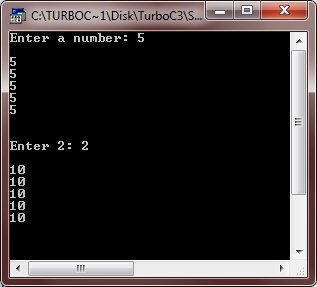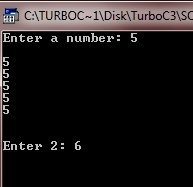Here is one more example for the complete understanding on the auto storage class of C.

```/* C Storage Class - The auto Storage Class */

#include<stdio.h>
#include<conio.h>
#include<stdlib.h>
void main()
{
int num1, num2, check;
clrscr();

num1 = rand();
num2 = rand();
printf("%d + %d = ", num1, num2);
scanf("%d", &check);
if(check == num1+num2)
{
auto int a, b, i;
printf("Enter any two number: ");
scanf("%d%d", &a, &b);
printf("\n");
for(i=0; i<5; i++)
{
printf("%d + %d = %d\tand\t%d - %d = %d", a, b, a+b, a, b, a-b);
printf("\n");
a++;
b++;
}
}

getch();
}```

Here is the sample run of this C program: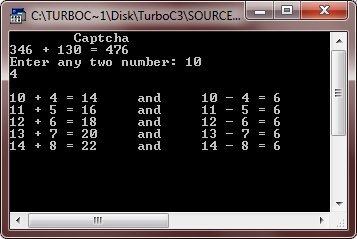When you enter wrong Captcha, then nothing will display as shown in this output.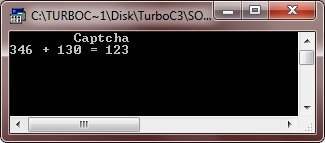If you use the variables, a, b, or i outside the their block, then an error occurred. In other words, the variables a, b, and i is unknown from outside that block where they are defined.

## C static Storage Class

The static storage class is used to create a permanent variable in a program. In other words, the static storage class simply tells the compiler to keep the local variable in existing during the life-time of the program. That is, when you apply the static modifier to a variable, then the compiler creates a permanent storage for this variable.

It is important to use a static variable for the creation of stand-alone functions because several types of routines must preserve a value between calls. Let's take some examples demonstrating the concept of static storage class in C. Let's start with first two program. The first one don't use static variable or the first program is without static variable. Whereas the second program is with the static variable to understand what is the use of static storage class in a program.

```/* C Storage Classes - The static Storage Class */

#include<stdio.h>
#include<conio.h>
void main()
{
int i, j;
clrscr();

for(i=0; i<5; i++)
{
for(j=0; j<5; j++)
{
int num = 5;
printf("%d\t", num);
num++;
}
printf("\n");
}

getch();
}```

Here is the sample output of this C program:Here is one more example. This program actually uses the static variable.

```/* C Storage Classes - The static Storage Class */

#include<stdio.h>
#include<conio.h>
void main()
{
int i, j;
clrscr();

for(i=0; i<5; i++)
{
for(j=0; j<5; j++)
{
static int num = 5;
printf("%d\t", num);
num++;
}
printf("\n");
}

getch();
}```

Here is the sample output of the above C program: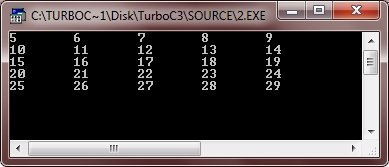Let's take another example.

```/* C Storage Classes - The static Storage Class */

#include<stdio.h>
#include<conio.h>
void main()
{
int j, check;
clrscr();

printf("Enter 2: ");
scanf("%d", &check);
if(check == 2)
{
static int num;
printf("Enter any number: ");
scanf("%d", &num);
printf("\nThe next 10 numbers are:\n");
num++;
for(j=0; j<10; j++)
{
printf("%d\n", num);
num++;
}
}

getch();
}```

Below is the sample run of this C program:Let's see, what happen, if you enter any number other than 2.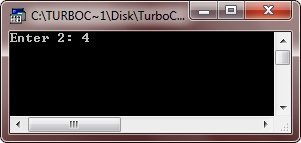Here is one more example.

```/* C Storage Class - The static Storage Class */

#include<stdio.h>
#include<conio.h>

void func(void);
static int count = 10;

void main()
{
clrscr();

while(count--)
{
func();
}

getch();
}

void func(void)
{
static int num = 10;
num++;

printf("num is %d and count is %d\n", num, count);
}```

Below is the sample run of the above C program: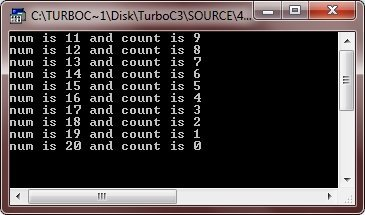## C register Storage Class

Before going to discuss about the register storage class. Let's first know that the register storage specifier originally applied only to the variables of type int, char, or pointer types.

The register specifier requested from the compiler to keep the value of a variable in a register of the CPU rather than in the memory (where normal variables are stored) for instant access to that variable. In other words, the register variables are used to perform faster access to the value of that variable. It meant that the operations on a register variable could occur much faster than no a normal variable because, as already told, the register variable was actually held in the CPU and didn't require the memory access to determine or modify its value.

The register variable is used to provide access to their value as fast as possible. Let's take an example using register variable.

```int int_pow(register int num, register int e)
{
register int temp;
temp = 1;

for(; e; e--)
{
temp = temp * num;
}

return temp;
}```

Here, in the above example, the variables num, e, and temp are declared as register variables because they are all used within the loop. The fact that the register variables are optimized for the speed makes. Here is the complete program for the complete understanding on the register storage class in C:

```/* C Storage Class - The register Storage Class */

#include<stdio.h>
#include<conio.h>

int int_pow(int, int);

void main()
{

int num, e, i;
clrscr();

printf("Enter a number and exponent: ");
scanf("%d%d", &num, &e);
printf("\n");
for(i=1; i<=e; i++)
{
if(i==e)
{
printf("%d", num);
}
else
{
printf("%d * ", num);
}
}

printf(" = %d", int_pow(num, e));

getch();
}

int int_pow(register int num, register int e)
{
register int temp;
temp = 1;

for(; e; e--)
{
temp = temp * num;
}
return temp;
}```

Here are the two sample run of the above C program.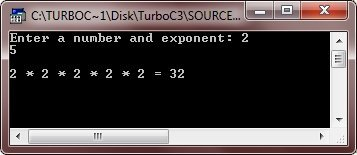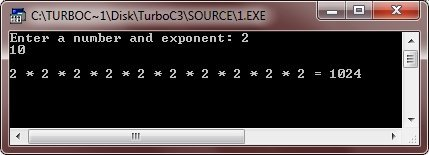Note - The register variable(s) has/have a maximum size equal to the register size.

Note - The compiler automatically transforms the register variables into non-register or normal variables when the limit is reached.

## C extern Storage Class

The extern storage class is simply used to give a reference of a global variable that is visible to all the program files. Here is a simple program demonstrating the extern storage class in C.

```/* C Storage Class - The extern Storage Class */

#include<stdio.h>
#include<conio.h>
void main()
{
extern int num1, num2;     // global variables
clrscr();

printf("%d\t%d", num1, num2);

getch();
}

// global variables definition of num1 and num2
int num1 = 10, num2 = 20;```

Here is the sample output of the above C program: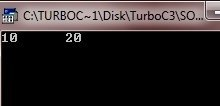Tools
Calculator